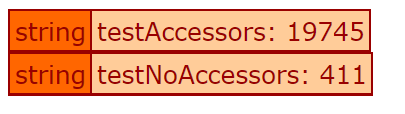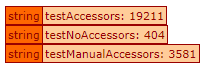# Size of implicit setters/getters in an object

We have been doing some analysis of an App recently, and found that many of our objects are taking up quite a bit of memory. As an example, one object is taking up 130MB when loaded into Application scope.

On investigation (using the very cool sizeof() method in Lucee) we have found that most of this is being made up by the implicit setter and getter methods. Each accessor is 1.4mb. So each property we add to a model adds 2.8mb to that object when loaded. This seems huge given what an accessor needs to do(?) … (read or write a field to a DB).

Is anyone able to tell me if this is normal? Or is there something wrong here that we need to look into further. Is there some kind of setting or configuration that can reduce the size of these accessors?

Jason

``````component accessors="true" {

property name="a" default="1";
property name="b" default="1";
property name="c" default="1";
property name="d" default="1";

public function init(){
return this;
}
}
``````
``````<cfscript>
a = new testAccessors();
dump("testAccessors: " & sizeOf(a));

a = new testNoAccessors();
dump("testNoAccessors: " & sizeOf(a));
</cfscript>
``````that’s 4,833.5 per property?

That is strange. What is stranger is that if you manually add all of the getters and setters it is still much less than the implicit accessors:

``````component {

property name="a" default="1";
property name="b" default="1";
property name="c" default="1";
property name="d" default="1";

public function init(){
return this;
}

public function geta(){
return variables.a;
}

public function getb(){
return variables.b;
}

public function getc(){
return variables.c;
}

public function getd(){
return variables.d;
}

public function seta(){
variables.a = arguments.a;
}

public function setb(){
variables.b = arguments.a;
}

public function setc(){
variables.c = arguments.a;
}

public function setd(){
variables.d = arguments.a;
}

}
``````Can you provide an example?

Thanks gents.

Here is the top few lines of my model:

``````component persistent="true" table="org" hint="Top level organisation" extends="coldbox.system.orm.hibernate.ActiveEntity"{

property name="SettingService" inject="model:SettingService" persistent="false";
property name="orm" inject="coldbox:plugin:ORMService" persistent="false";

property name="id" fieldtype="id" generator="native";
property name="name" ormtype="string" length="150";
property name="name_abbr" ormtype="string" length="20";
property name="domain" ormtype="string" length="100";
property name="contactName" ormtype="string" length="100";
property name="contactPhone" ormtype="string" length="100";
property name="contactFax" ormtype="string" length="100";
property name="contactEmail" ormtype="string" length="100";
``````

Thun dumping out size of each key (where greater than 1400000 bytes):

``````<cfscript>
for (key in structKeyList(Application.org)) {

size = sizeof( evaluate('Application.org.#key#') );

if (size > 1400000) {
dump(key);
dump( size )
writeOutput('<hr>')
}

}

abort;
</cfscript>
``````

And here is debug output

Also I have tried it without extending teh Coldbox Active Entity and it made no notable reduction in size.

I’ve created an issue to investigate this [LDEV-3335] implicit setters/getters in an object increase size - Lucee

could you provide a whole test case, rather that just a snippet?